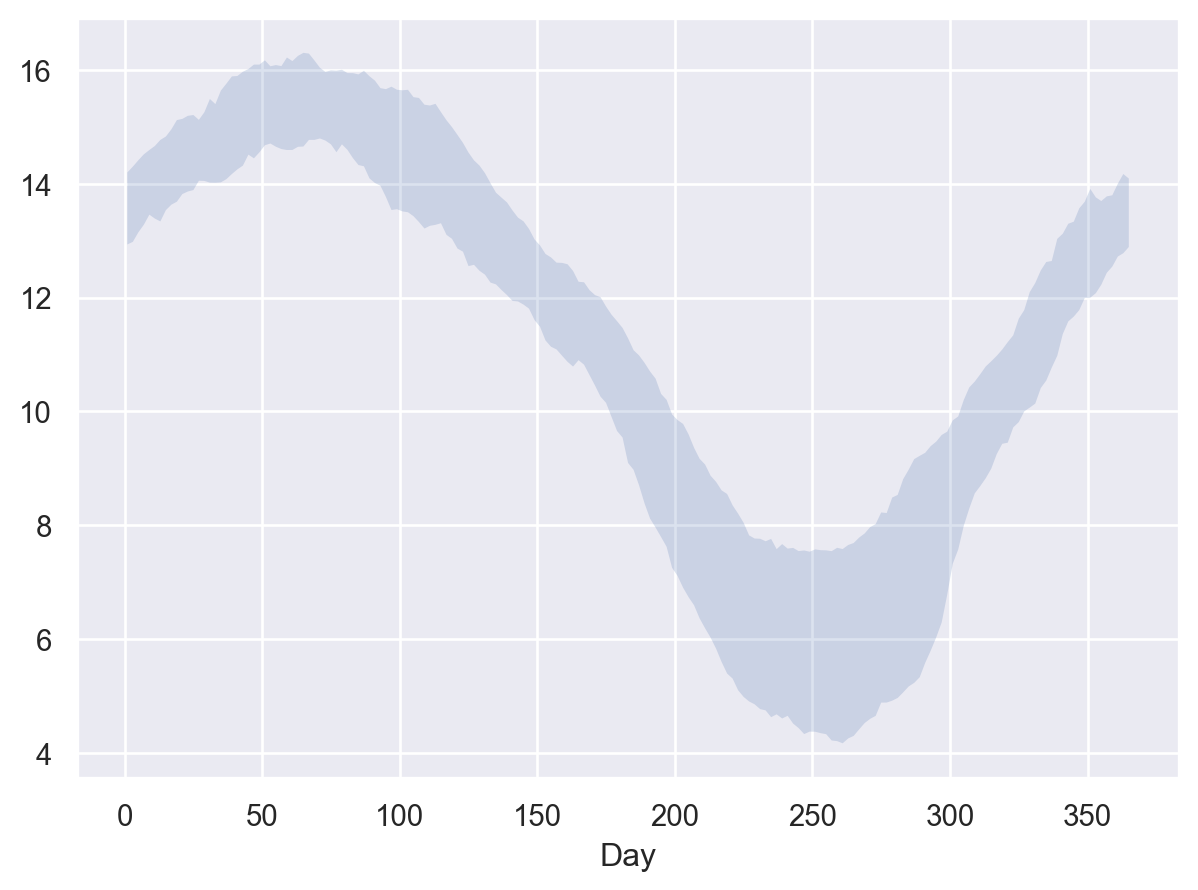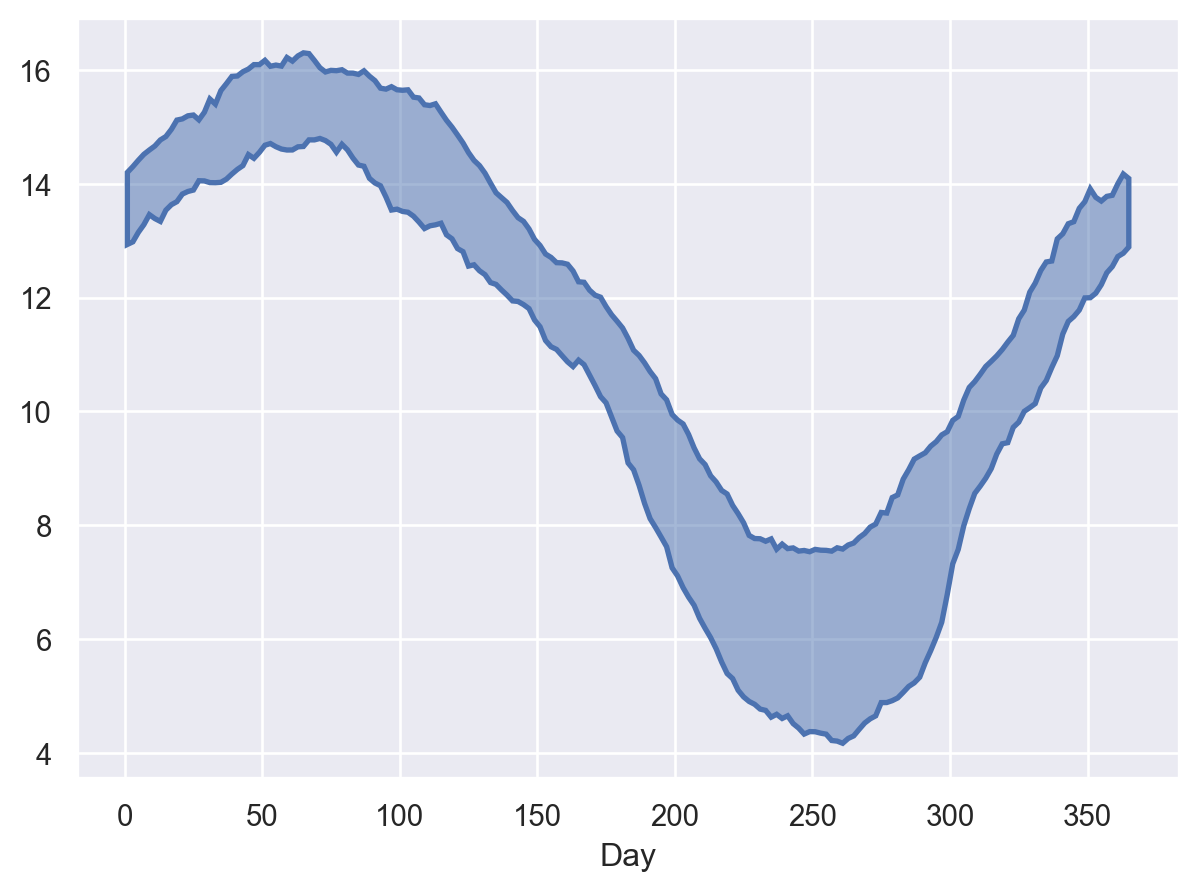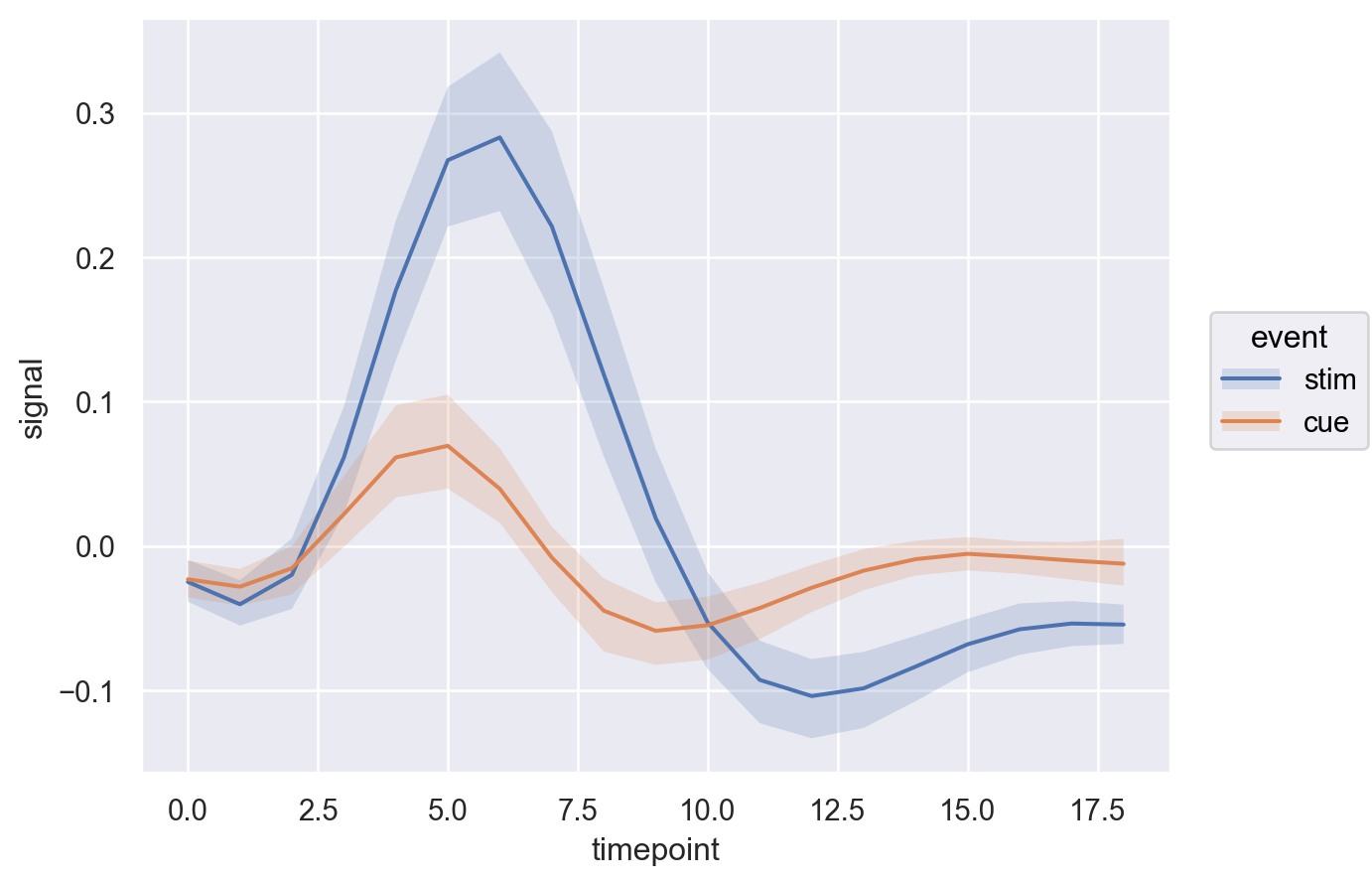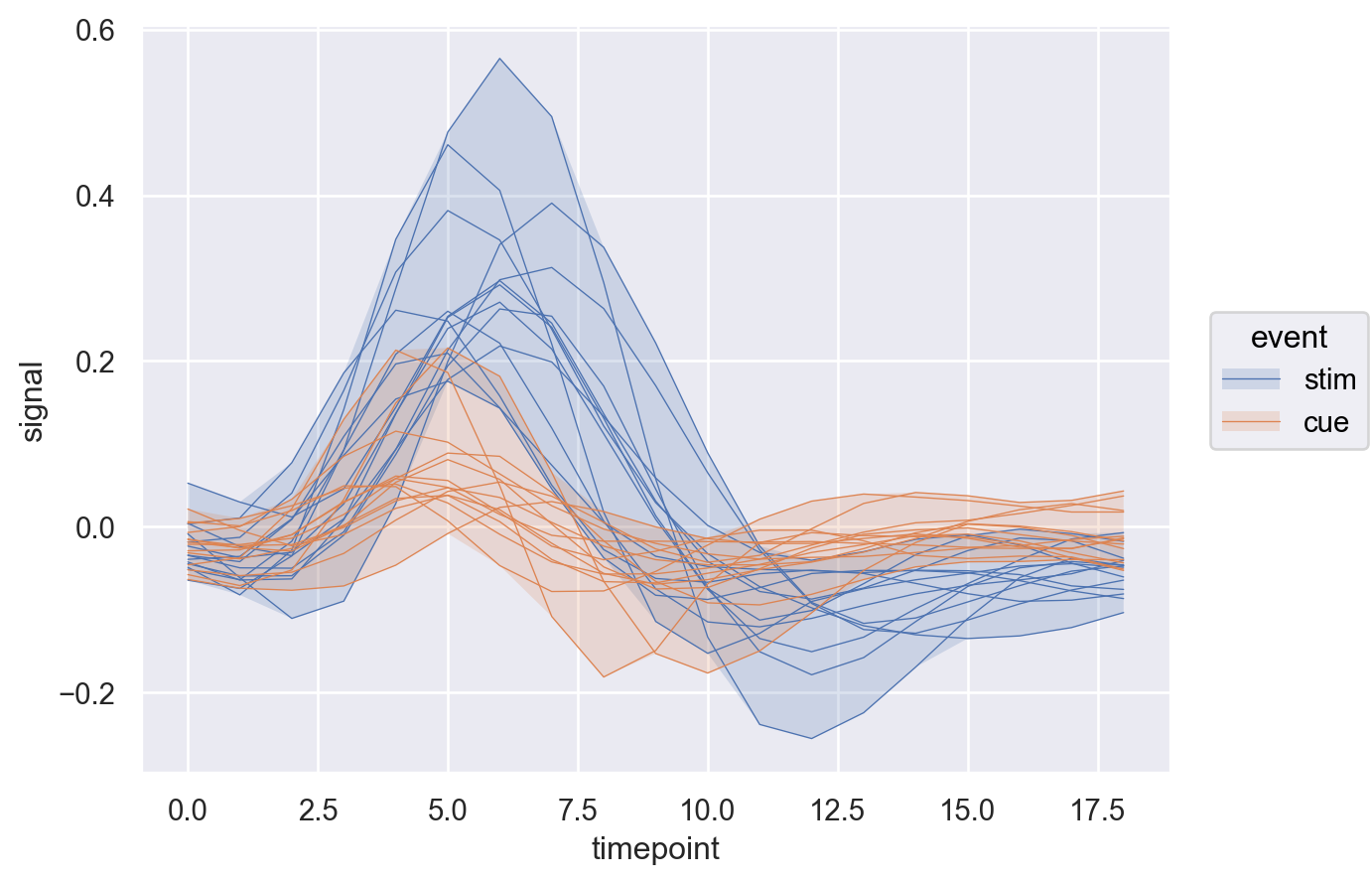# seaborn.objects.Band#

class seaborn.objects.Band(artist_kws=<factory>, color=<'C0'>, alpha=<0.2>, fill=<True>, edgecolor=<depend:color>, edgealpha=<1>, edgewidth=<0>, edgestyle=<'-'>)#

A fill mark representing an interval between values.

This mark defines the following properties:

`Area`

A fill mark drawn from a baseline to data values.

Examples

The mark fills between pairs of data points to show an interval on the value axis:

```p = so.Plot(seaice, x="Day", ymin="1980", ymax="2019")
```By default it draws a faint ribbon with no edges, but edges can be added:

```p.add(so.Band(alpha=.5, edgewidth=2))
```The defaults are optimized for the main expected usecase, where the mark is combined with a line to show an errorbar interval:

```(
so.Plot(fmri, x="timepoint", y="signal", color="event")```(